# AP Calculus BC Practice Test 3

### Test Information9 questions18 minutes

Calculator Disallowed

1. The length of the curve determined by x = 3t and y = 2t2 from t = 0 to t = 9 is

2. If a particle moves in the xy-plane so that at time t > 0 its position vector is (et2, e-t3), then its velocity vector at time t = 3 is

3. The graph of f(x) =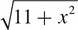has a point of inflection at

4. Find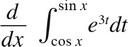5.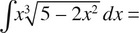6. A rectangle is to be inscribed between the parabola y = 4 - x2 and the x-axis, with its base on the x-axis. A value of x that maximizes the area of the rectangle is

7.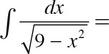8. Find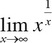.

9. What is the sum of the Maclaurin series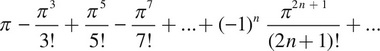?